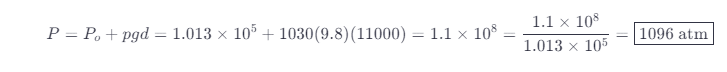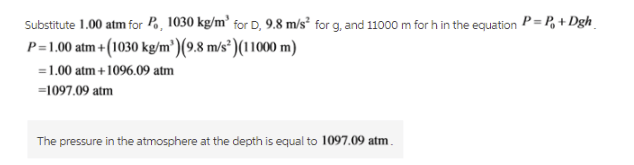# The Deepest Point In The Ocean Is 11 Km Below Sea Level, Deeper Than Mt. Everest Is Tall.

We thoroughly check each answer to a question to provide you with the most correct answers. Found a mistake? Let us know about it through the REPORT button at the bottom of the page.

The deepest point in the ocean is 11 km below sea level, deeper than Mt. Everest is tall. What is the pressure in atmospheres at this depth?

The equation for pressure is given by:The density of the water is equal to 1000 kg/m^3. But we have to calculate the depth of the point in the ocean. The ocean contains saltwater so, we have to use the density of the saltwater.